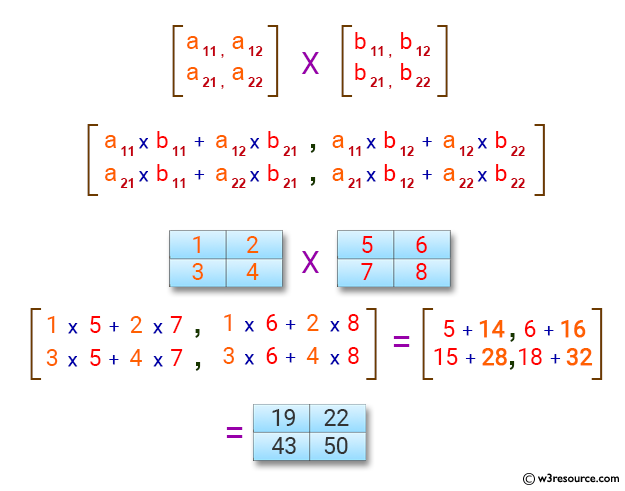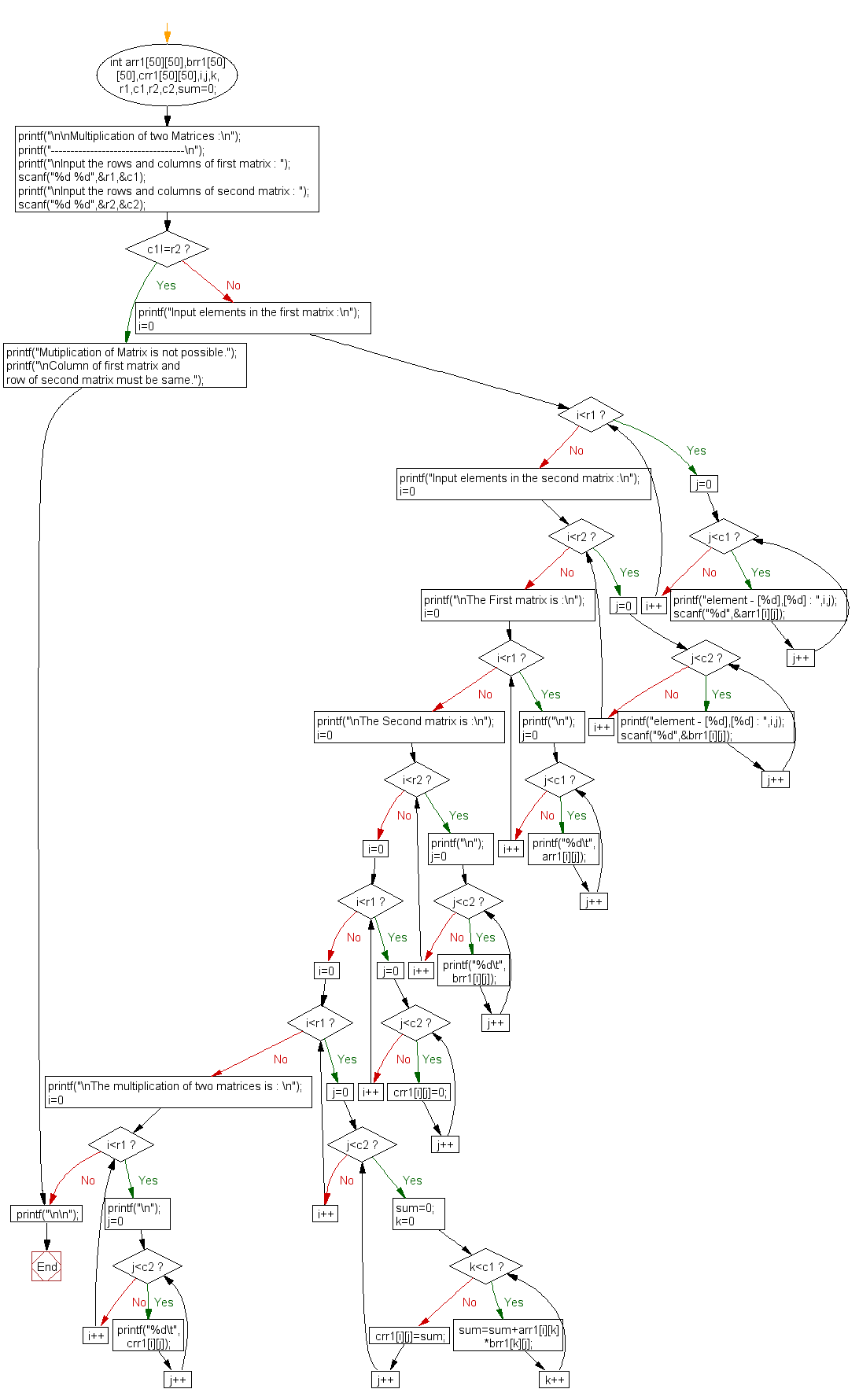﻿ C exercises: Multiplication of two Matrices - w3resource# C Exercises: Multiplication of two Matrices

## C Array: Exercise-21 with Solution

Write a program in C for multiplication of two square Matrices.

Pictorial Presentation:Sample Solution:

C Code:

``````#include <stdio.h>

void main()
{
int arr1,brr1,crr1,i,j,k,r1,c1,r2,c2,sum=0;

printf("\n\nMultiplication of two Matrices :\n");
printf("----------------------------------\n");

printf("\nInput the rows and columns of first matrix : ");
scanf("%d %d",&r1,&c1);
printf("\nInput the rows and columns of second matrix : ");
scanf("%d %d",&r2,&c2);
if(c1!=r2){
printf("Mutiplication of Matrix is not possible.");
printf("\nColumn of first matrix and row of second matrix must be same.");
}
else
{
printf("Input elements in the first matrix :\n");
for(i=0;i<r1;i++)
{
for(j=0;j<c1;j++)
{
printf("element - [%d],[%d] : ",i,j);
scanf("%d",&arr1[i][j]);
}
}
printf("Input elements in the second matrix :\n");
for(i=0;i<r2;i++)
{
for(j=0;j<c2;j++)
{
printf("element - [%d],[%d] : ",i,j);
scanf("%d",&brr1[i][j]);
}
}
printf("\nThe First matrix is :\n");
for(i=0;i<r1;i++)
{
printf("\n");
for(j=0;j<c1;j++)
printf("%d\t",arr1[i][j]);
}

printf("\nThe Second matrix is :\n");
for(i=0;i<r2;i++)
{
printf("\n");
for(j=0;j<c2;j++)
printf("%d\t",brr1[i][j]);
}
//multiplication of matrix
for(i=0;i<r1;i++)
for(j=0;j<c2;j++)
crr1[i][j]=0;
for(i=0;i<r1;i++)    //row of first matrix
{
for(j=0;j<c2;j++)    //column of second matrix
{
sum=0;
for(k=0;k<c1;k++)
sum=sum+arr1[i][k]*brr1[k][j];
crr1[i][j]=sum;
}
}
printf("\nThe multiplication of two matrices is : \n");
for(i=0;i<r1;i++)
{
printf("\n");
for(j=0;j<c2;j++)
{
printf("%d\t",crr1[i][j]);
}
}
}
printf("\n\n");
}
```
```

Sample Output:

```Multiplication of two Matrices :
----------------------------------

Input the rows and columns of first matrix : 2
2

Input the rows and columns of second matrix : 2
2
Input elements in the first matrix :
element - , : 1
element - , : 2
element - , : 3
element - , : 4
Input elements in the second matrix :
element - , : 5
element - , : 6
element - , : 7
element - , : 8

The First matrix is :

1       2
3       4
The Second matrix is :

5       6
7       8
The multiplication of two matrices is :

19      22
43      50
```

Flowchart:C Programming Code Editor:

Improve this sample solution and post your code through Disqus.

What is the difficulty level of this exercise?

﻿

## C Programming: Tips of the Day

Where is the C auto keyword used?

auto is a modifier like static. It defines the storage class of a variable. However, since the default for local variables is auto, you don't normally need to manually specify it.

Ref : https://bit.ly/3yzwC9r